前言

https://www.cnblogs.com/czaoth/p/6912073.html

前置芝士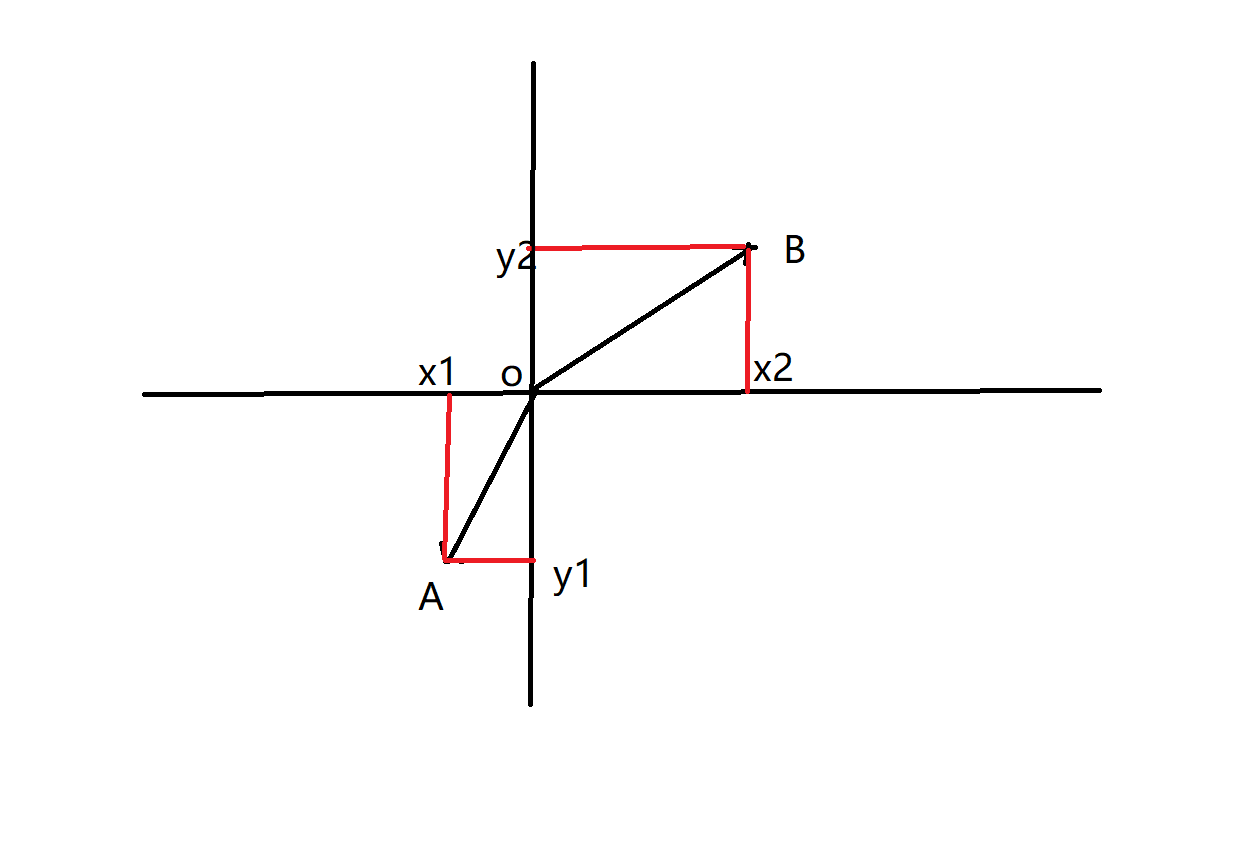$\vec{a}\times \vec{b}=x1\times y2 - x2\times y1$

关于二维凸包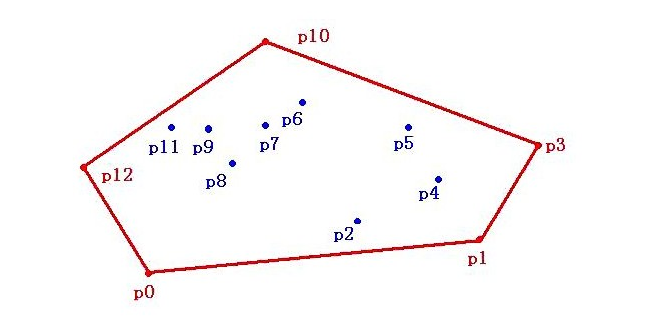分治法$O(nlogn)$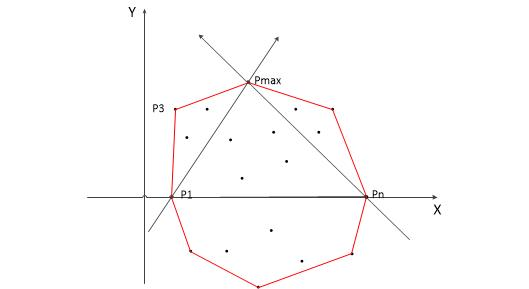Jarvis步进法$O(NH)$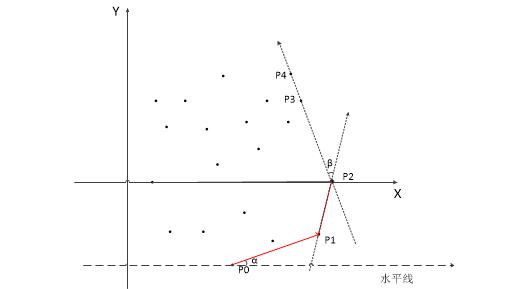Graham Scan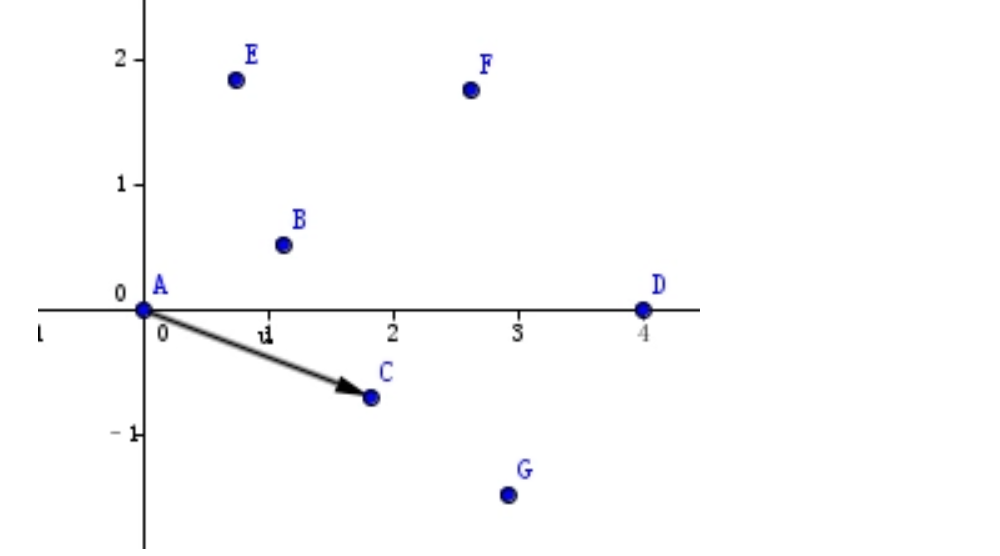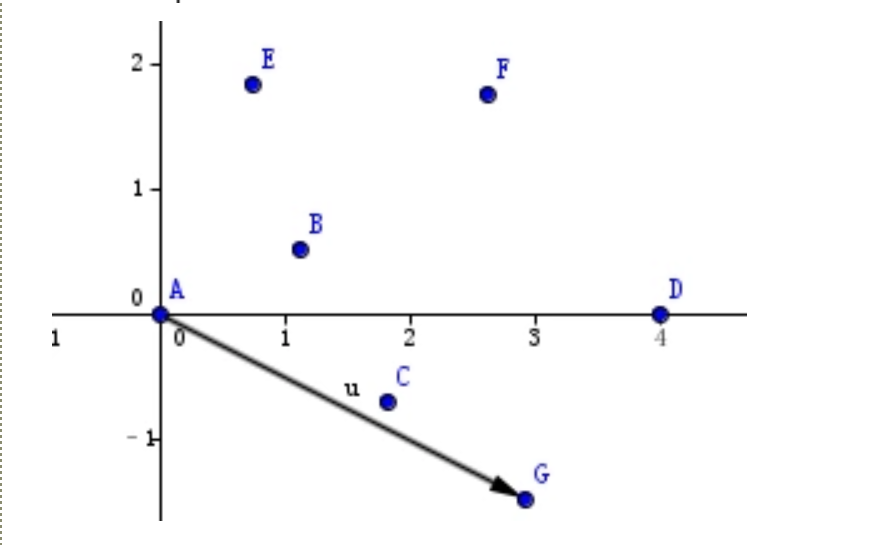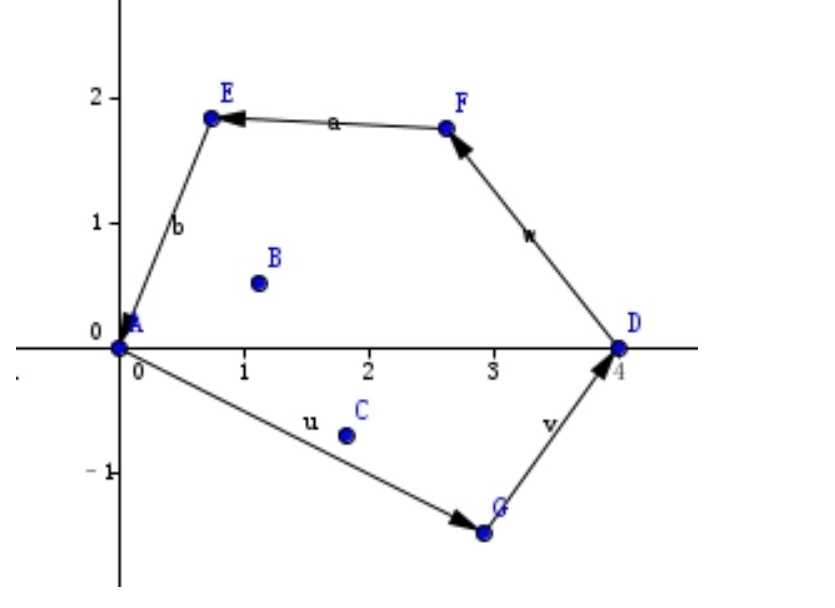#include <bits/stdc++.h>
#define db double
#define N 10005
using namespace std;
template <typename T> T sqr(T x) {return x * x;}
struct Point {
db x, y;
}p[N], s[N];
db dist(Point p1, Point p2) {//计算距离
return sqrt(sqr(p1.x - p2.x) + sqr(p1.y - p2.y));
}
bool cmp(Point p1, Point p2) {//排序
return (p1.x == p2.x)? (p1.y < p2.y): (p1.x < p2.x);
}
db cross(Point p1, Point p2) {//叉积
return p1.x * p2.y - p1.y * p2.x;
}
db check(Point p1, Point p2, Point p3, Point p4) {//查看两个线段的向量的夹角（逆时针）
return cross((Point){p2.x - p1.x, p2.y - p1.y},(Point){p4.x - p3.x, p4.y - p3.y}) <= 0;
}
int n, tot;
db ans = 0;
int main() {
scanf("%d", &n);
for (int i = 1; i <= n; i ++) scanf("%lf%lf", &p[i].x, &p[i].y);
sort(p + 1, p + 1 + n, cmp);
tot = 0;
for (int i = 1; i <= n; i ++) {//计算上凸包
while (tot > 1 && check(s[tot], s[tot - 1], s[tot], p[i])) -- tot;
s[++ tot] = p[i];
}
int tmp = tot;
for (int i = n - 1; i >= 1; i --) {//下凸包
while (tot > tmp && check(s[tot], s[tot - 1], s[tot], p[i])) -- tot;
s[++ tot] = p[i];
}
for (int i = 2; i <= tot; i ++) ans += dist(s[i], s[i - 1]);
printf("%.2lf\n", ans);
return 0;
}

• 找到最下面的一个点，如果存在纵坐标相同，取最左面的点。取这个点作为原点。
• 把p0同点集中其他各点用线段连接，并计算这些线段与水平线的夹角，然后按夹角从小到大排序（夹角范围为 [0, 180)度），如果存在夹角相同的点，取最远点其余点直接删除。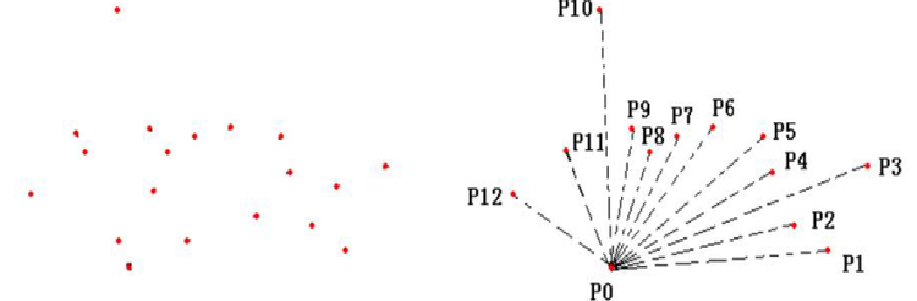• 创建一个栈，将p0,p1,p2三点放入栈中，之后依次遍历p3~pn各点，若栈顶的两个点和当前的点pi这三点连线的方向向顺时针方向偏转，表明栈顶的点是一个凹陷处，应删除，则栈顶元素出栈。并将pi压入栈中。如果向逆时针方向偏转，则直接压入栈中。
• 重复上述过程，直到遍历完成，在栈中的点就是凸包上的顶点。（结果图是最上边的图）
• 不要忘记加最后一个起点。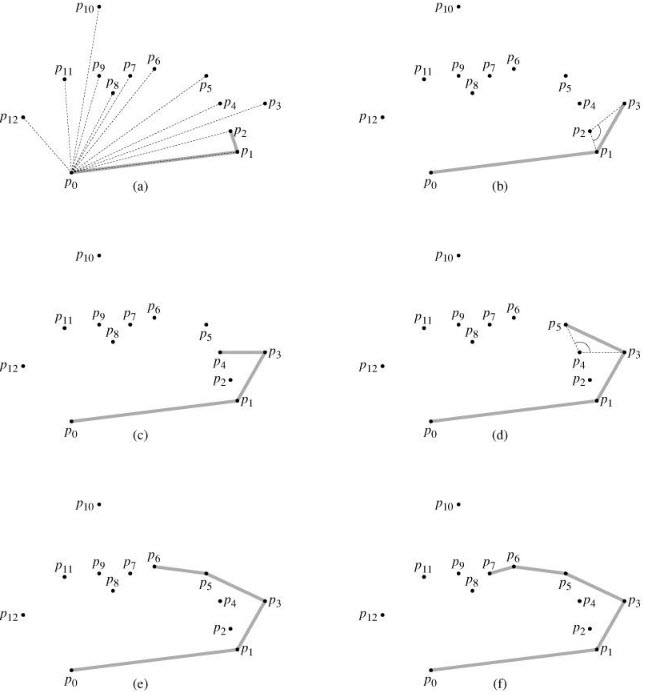动态图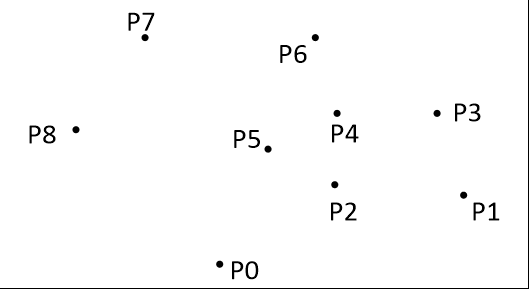#include <bits/stdc++.h>
#define ll long long
#define db double
#define ms(a, b) memset(a, b, sizeof(a))
#define inf 0x3f3f3f3f
#define N 10005
using namespace std;
template <typename T>
inline void read(T &x) {
x = 0; T fl = 1; char ch = 0;
for (; ch < '0' || ch > '9'; ch = getchar())
if (ch == '-') fl = -1;
for (; ch >= '0' && ch <= '9'; ch = getchar())
x = (x << 1) + (x << 3) + (ch ^ 48);
x *= fl;
}
template <typename T> T sqr(T x) {return x * x;}
struct node {
db x, y;
}p[N], S[N];
int n, top;
db ans = 0.0;
db X(node a1, node a2, node b1, node b2) {
return (a2.x - a1.x) * (b2.y - b1.y) - (b2.x - b1.x) * (a2.y - a1.y);
}
bool cmp(node a, node b) {
return (a.x == b.x) ? (a.y < b.y): (a.x < b.x);
}
db dis(node a, node b) {
return sqrt(sqr(a.x - b.x) + sqr(a.y - b.y));
}
bool cmp2(node p1, node p2) {
db tmp = X(p, p1, p, p2);
if (tmp > 0)  return 1;
if (tmp == 0 && dis(p, p1) < dis(p, p2)) return 1;
return 0;
}
int main() {
for (int i = 1; i <= n; i ++)
scanf("%lf%lf", &p[i].x, &p[i].y);
sort(p + 1, p + 1 + n, cmp);
S[++ top] = p;
sort(p + 2, p + n + 1, cmp2);
for (int i = 2; i <= n; i ++) {
while (top > 1 && X(S[top - 1], S[top], S[top], p[i]) <= 0) top --;
S[++ top] = p[i];
}
ans = dis(S, S[top]);
for (int i = 2; i <= top; i ++) ans += dis(S[i - 1], S[i]);
printf("%.2lf\n", ans);
return 0;
}
posted @ 2019-03-31 21:13 chhokmah 阅读(...) 评论(...) 编辑 收藏